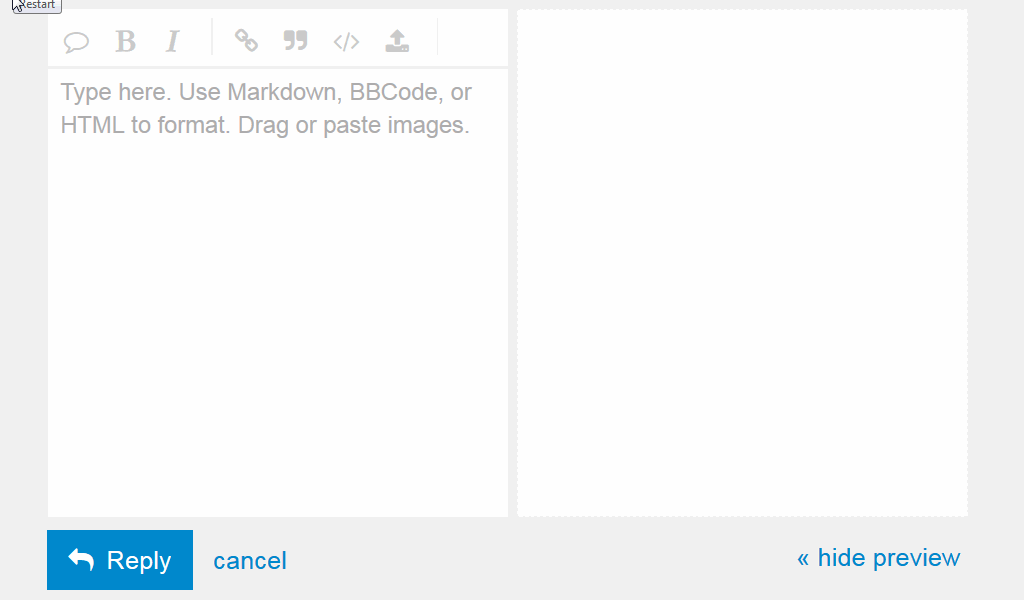# Find the Longest Word

Find the Longest Word
0

#1

I wrote such code and i can’t understand why my loop doesn’t work (it shows check mark only for example “Google do a barrel roll” where the the first word is longest. i would be glad any advice before i will go to see someone’s code.

Your code so far

``````function findLongestWord(str) {
var arr = str.split(' ');

for (var n = 0;n <=arr.length; n++) {
var max = 0;
if (arr[n].length >= arr[n+1].length) {
if (arr[n].length > max)
max = arr[n].length;

}
else {
if (arr[n+1].length > max)
max = arr[n+1].length;

}
return max;
}

}

findLongestWord("Google do a barrel roll");
``````

#2

Your code only makes it one iteration, before you return max, because your return statement is inside the for loop. Once a return statement is executed, the function is exited immediately and does not wait to complete for loop iteration.

Moving the return max will not give you a passing solution, but will get you past the first word.

HINT: The following is going to be a problem:

``````arr[n+1].length
``````

#3

Thanks randelldawson. I’m glad that i needn’t to see finished works
I did it with yout remark though still not absolutely sure why it worked =) Last thing i changed was < instead of <= and all worked

``````function findLongestWord(str) {
var arr = str.split(' ');
var max = 0;
for (var n = 0; n <arr.length; n++) {

if (arr[n].length > max) {
max = arr[n].length;
}

}
return max;
}

findLongestWord("Google do a barrel roll");
``````

#4

Other than the ‘return’ problem, I noticed :

In the first block of code you had placed the ‘var max = 0’ inside the for loop where it would be set back to ‘0’ for each iteration.

In the code modifications where it worked, you have moved ‘var max = 0;’ out of the loop so it can keep track of the longest word encountered without being reset each time.

With max being reset on each iteration I think the effect would have been to return the length of the last word tested every time?

#5

Yes, exactly, i played with this variable also

#6

I’ve edited your post for readability. When you enter a code block into the forum, remember to precede it with a line of three backticks and follow it with a line of three backticks to make easier to read. See this post to find the backtick on your keyboard. The “preformatted text” tool in the editor (`</>`) will also add backticks around text.